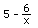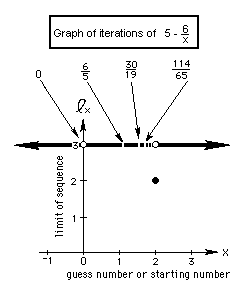### Solve the quadratic equation x2 - 5x + 6 = 0 to get x=5-6/x. Iterate the function 5-6/x and graph the starting number vs the limit of the infinite sequence that is formed

2b.We'll iterate the functionstarting with different numbers, then graph the limits of the infinite sequences we get.
Starting number 1. Putting 1->x, we get 5-(6/1)=-1. Putting this output number -1 in for x now, we get 5-(6/-1) = 11. Putting 11 -> x we get 5-(6/11)= 4.45..
So we get this infinite sequence:
1, -1, 11, 4.45.., 3.653..., 3.3575..., 3.2129..., ...which goes to 3 as the limit.
Starting no. -100, 5.06, 3.81422..., 3.4269..., 3.2491...,3.1533..., ...which goes to 3 as the limit.Notice that starting with 2 we get a constant sequence 2, 2, 2, ... On the graph 2 goes to 2.
All other starting numbers lead to an infinite sequence which goes to 3 as the limit.., except an infinite number of starting numbers like zero, and 6/5 that at some point make the denominator go to 0 and make the fraction 'blow up' and thus has no answer. These numbers put a hole in the graph. Can you find a rule for these numbers that make the denominato 0? What is the limit of the sequence of these numbers?
To order Don's materials
Back to Ch. 8, part 2- iteration
Mathman home